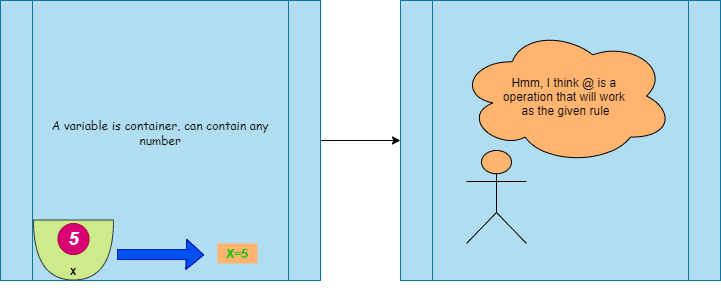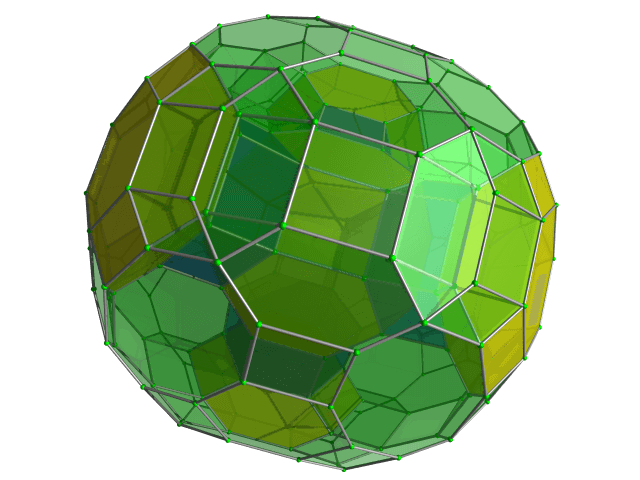Categories

# Ratio and proportion AMC 8, 2010 Problem 2

Try this beautiful problem from AMC 8. It involves the concept of a variable, binary operation and ratio-proportion. We provide sequential hints so that you can try the problem.

## Competency in Focus:Ratio and proportion.

This problem from American Mathematics contest (AMC 8, 2010) is based on the concept of ratio and proportion and binary composition .

## First look at the knowledge graph.## Next understand the problem

If $a @ b = \frac{a\times b}{a+b}$ for $a,b$ positive integers, then what is $5 @10$? $\textbf{(A)}\ \frac{3}{10} \qquad\textbf{(B)}\ 1 \qquad\textbf{(C)}\ 2 \qquad\textbf{(D)}\ \frac{10}{3} \qquad\textbf{(E)}\ 50$
##### Source of the problem
American Mathematical Contest 2010, AMC 8  Problem 2

### Ratio and Proportion

2/10
##### Suggested Book

Do you really need a hint? Try it first!
Take a look at the knowledge graph first
Substitute $a \textbf{ by } 5 \textbf{ and } b \text{ by } 10$
$5@10=\frac{5\times10}{5+10}=\frac{50}{15}=\frac{10}{3}$

## AMC - AIME Program

AMC - AIME - USAMO Boot Camp for brilliant students. Use our exclusive one-on-one plus group class system to prepare for Math Olympiad## External Tangent | AMC 10A, 2018 | Problem 15

Try this beautiful Problem on geometry based on circle from AMC 10A, 2018. Problem-15. You may use sequential hints to solve the problem.

## Dice Problem | AMC 10A, 2014| Problem No 17

Try this beautiful Problem on Probability from AMC 10A, 2014. Problem-17, You may use sequential hints to solve the problem.

## Problem on Curve | AMC 10A, 2018 | Problem 21

Try this beautiful Problem on Co-ordinate geometry from AMC 10A, 2018. Problem-21, You may use sequential hints to solve the problem.

## Right-angled Triangle | AMC 10A, 2018 | Problem No 16

Try this beautiful Problem on triangle from AMC 10A, 2018. Problem-16. You may use sequential hints to solve the problem.

## Finding Greatest Integer | AMC 10A, 2018 | Problem No 14

Try this beautiful Problem on Algebra from AMC 10A, 2018. Problem-14, You may use sequential hints to solve the problem.

## Length of the crease | AMC 10A, 2018 | Problem No 13

Try this beautiful Problem on triangle from AMC 10A, 2018. Problem-13. You may use sequential hints to solve the problem.

## Right-angled shaped field | AMC 10A, 2018 | Problem No 23

Try this beautiful Problem on triangle from AMC 10A, 2018. Problem-23. You may use sequential hints to solve the problem.

## Area of region | AMC 10B, 2016| Problem No 21

Try this beautiful Problem on Geometry on Circle from AMC 10B, 2016. Problem-20. You may use sequential hints to solve the problem.

## Coin Toss Problem | AMC 10A, 2017| Problem No 18

Try this beautiful Problem on Probability from AMC 10A, 2017. Problem-18, You may use sequential hints to solve the problem.

## GCF & Rectangle | AMC 10A, 2016| Problem No 19

Try this beautiful Problem on Geometry on Rectangle from AMC 10A, 2010. Problem-19. You may use sequential hints to solve the problem.

This site uses Akismet to reduce spam. Learn how your comment data is processed.